# Triangle Problems

#### Number of problems found: 1282

• Trigonometric functionsIn the right triangle is: tg α= frac(2) 1 Find the value of s and c: sin α= (s)/(√ 5) cos α= (c)/(√ 5)
• Diagonal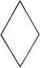Can be a diagonal of diamond twice longer than it side?
• Diagonals of the rhombus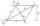Calculate height of rhombus whose diagonals are 12 cm and 19 cm.
• 3-bracket 3Two angles in a triangle are 90° and 60°. Has triangle at least two equal sides?
• 3-bracket 2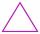May be the smallest angle in the triangle greater than 70°?
• 3-bracketMay be the largest angle in the triangle less than 20°?
• DiagonalCan a rhombus have the same length diagonal and side?
• Chord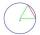Point on the circle is the end point of diameter and end point of chord length of radius. What angle between chord and diameter?
• ChordIn a circle with radius r=60 cm is chord 4× longer than its distance from the center. What is the length of the chord?
• Is right?Is a triangle with sides 51, 56, and 77 right triangle?
• BoxCalculate the angle between box base 9 x 14 and body diagonal length 18.
• Median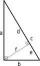In the right triangle are sides a=96 dm b=31 dm. Calculate the length of the medians tc to the hypotenuse.
• Isosceles triangle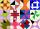Calculate the perimeter of isosceles triangle with arm length 73 cm and base length of 48 cm.
• AnglesThe triangle is one outer angle 158°54' and one internal angle 148°. Calculate the other internal angles of a triangle.
• V-belt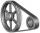Calculate the length of the belt on pulleys with diameters of 105 mm and 393 mm at shaft distance 697 mm.
• Track arc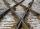Two straight tracks is in an angle 74°. They will join with circular arc with radius r=1127 m. How long will be arc connecting these lines (L)? How far is the center point of arc from track crossings (x)?
• Heron backlawCalculate missing side in a triangle with sides 17 and 34 and area 275.
• Sphere cutsAt what distance from the center intersects sphere with radius R = 56 plane, if the cut area and area of the main sphere circle is in ratio 1/2.
• Equilateral triangleCalculate the side of an equilateral triangle if its area is 892 mm2.
• Right triangleRight triangle legs has lengths 630 mm and 411 dm. Calculate the area of this triangle.

Do you have an exciting math question or word problem that you can't solve? Ask a question or post a math problem, and we can try to solve it.

We will send a solution to your e-mail address. Solved examples are also published here. Please enter the e-mail correctly and check whether you don't have a full mailbox.